# JAC Class 10 Maths Solutions Chapter 6 Triangles Ex 6.2

Jharkhand Board JAC Class 10 Maths Solutions Chapter 6 Triangles Ex 6.2 Textbook Exercise Questions and Answers.

## JAC Board Class 10 Maths Solutions Chapter 6 Triangles Exercise 6.2

Question 1.
In the given figures (1) and (2), DE || BC. Find EC in (1) and AD in (2).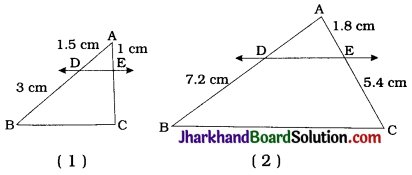1. Line DE is parallel to side BC of AABC and it intersects AB and AC at D and E respectively.
∴ $$\frac{AD}{DB}=\frac{AE}{EC}$$ (Theorem 6.1)
∴ $$\frac{1.5}{3}=\frac{1}{EC}$$
∴ EC = $$\frac{1 \times 3}{1.5}$$
∴ EC = 2cm

2. Line DE is parallel to side BC of ΔABC and it intersects AB and AC at D and E respectively.
∴ $$\frac{AD}{DB}=\frac{AE}{EC}$$ (Theorem 6.1)
∴ $$\frac{AD}{7.2}=\frac{1.8}{5.4}$$
∴ AD = $$\frac{1.8 \times 7.2}{5.4}$$Question 2.
E and F are points on the sides PQ and PR respectively of a PQR. For each of the following cases, state whether EF || QR
1. PE = 3.9 cm, EQ = 3 cm, PF = 3.6 cm and FR = 2.4 cm
2. PE = 4 cm, QE = 4.5 cm, PF = 8 cm and RF = 9 cm
3. Pg = 1.28 cm, PR = 2.56 cm, PE = 0.18 cm and PF = 0.36 cm
E and F are points on the sides PQ and PR respectively of ΔPQR.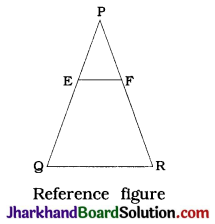1. Here, $$\frac{\mathrm{PE}}{\mathrm{EQ}}=\frac{3.9}{3}=\frac{1.3}{1}$$ and
$$\frac{\mathrm{PF}}{\mathrm{FR}}=\frac{3.6}{2.4}=\frac{1.5}{1}$$
So, $$\frac{PE}{EQ}$$ = $$\frac{PF}{FR}$$
Hence, by the converse of BPT, EF is not parallel to QR.

2. Here, $$\frac{\mathrm{PE}}{\mathrm{QE}}=\frac{4}{4.5}=\frac{8}{9}$$ and $$\frac{PF}{RF}$$ = $$\frac{8}{9}$$
So, $$\frac{PE}{QE}$$ = $$\frac{PF}{RF}$$
Hence, by the converse of BPT, EF || QR.

3. Here, $$\frac{\mathrm{PE}}{\mathrm{PQ}}=\frac{0.18}{1.28}=\frac{9}{64}$$ and
$$\frac{PF}{PR}=\frac{0.36}{2.56}=\frac{9}{64}$$
So, $$\frac{PE}{PQ}$$ = $$\frac{PF}{PR}$$
Hence, by theorem 6.2, EF || QR.

Question 3.
In the given figure, if LM || CB and LN || CD, prove that $$\frac{AM}{AB}$$ = $$\frac{AN}{AD}$$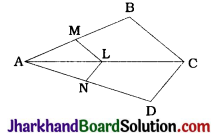In ΔABC, LM || CB and LM intersects AB and AC at M and L respectively.
∴ $$\frac{AM}{AB}=\frac{AL}{AC}$$(BPT) ……….(1)
In ΔACD, LN || CD and LN intersects AC and AD at L and N respectively.
∴ $$\frac{AL}{AC}=\frac{AN}{AD}$$(BPT) ……….(2)
From (1) and (2), $$\frac{AM}{AB}=\frac{AN}{AD}$$Question 4.
In the given figure, DE || AC and DF || AE.
Prove that $$\frac{AM}{AB}$$ = $$\frac{AN}{AD}$$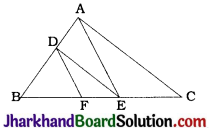In ΔABE, DF || AE and DF intersects AB and BE at D and F respectively.
∴ $$\frac{BF}{FE}=\frac{BD}{DA}$$ (BPT) …………(1)
In ΔABC, DE || AC and DE intersects BA and BC at D and E respectively.
∴ $$\frac{BD}{DA}=\frac{BE}{EC}$$ …………(2)
From (1) and (2), $$\frac{BF}{FE}=\frac{BE}{EC}$$

Question 5.
In the given figure, DE || OQ and DF || OR. Show that EF || QR.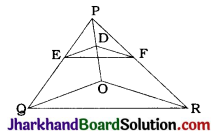In ΔPQO, DE || OQ
∴ $$\frac{PE}{EQ}=\frac{PD}{DO}$$ (BPT) …………(1)
In ΔPOR, DF || OR
∴ $$\frac{PD}{DO}=\frac{PF}{FR}$$ (BPT) …………(2)
From (1) and (2), $$\frac{PE}{EQ}=\frac{PF}{FR}$$
Now, line EF intersects sides PQ and PR of ΔPQR at E and F respectively and $$\frac{PE}{EQ}=\frac{PF}{FR}$$
Hence, by converse of BPT, EF || QR.

Question 6.
In the given figure, A, B and C are points on OP, OQ and OR respectively such that AB || PQ and AC || PR. Show that BC || QR.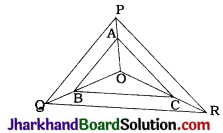In ΔOPQ, AB || PQ and AB intersects OP and OQ at A and B respectively.
∴ $$\frac{OA}{AP}=\frac{OB}{BQ}$$ (BPT) …………(1)
In ΔOPR, AC || PR and AC intersects OP and OR at A and C respectively.
∴ $$\frac{OA}{AP}=\frac{OC}{CR}$$ (BPT) …………(2)
From (1) and (2), $$\frac{OB}{BQ}=\frac{OC}{CR}$$
In ΔOQR, BC intersects OQ and OR at B and C respectively such that $$\frac{OB}{BQ}=\frac{OC}{CR}$$
∴ BC || QR (Converse of BPT)Question 7.
Using theorem 6.1, prove that a line drawn through the mid-point of one side of a triangle parallel to another side bisects the third side. (Recall that you have proved it in Class IX).
Given:
In ΔABC, P is the mid-point of side AB. Through P a line is drawn parallel to BC to intersect AC at Q.
To prove : Q is the mid-point of AC.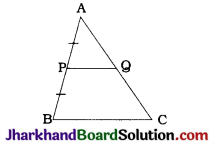Proof: In ΔABC, P and Q lie on AB and AC respectively such that PQ || BC.
∴ $$\frac{AP}{PB}=\frac{AQ}{QC}$$ (Theorem 6.1) ………(1)
Now, P is the mid-point of AB.
∴ AP = PB
∴ $$\frac{AP}{PB}$$ = 1 ………(2)
From (1) and (2).
$$\frac{AP}{PB}$$ = 1
∴ AQ = QC
∴ Q is the mid-point of AC.
Note: In Class IX, this was proved with the help of properties of a parallelogram.

Question 8.
Using theorem 6.2, prove that the line joining the mid-points of any two sides of a triangle is parallel to the third side. (Recall that you have done it in Class IX).
Given: In ΔABC, P and Q are the mid-points of AB and AC respectively.
To prove : PQ || BC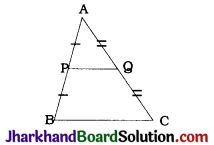Proof: Here, P is the mid-point of AB.
∴ AP = PB
∴ $$\frac{AP}{PB}$$ = 1 ……………(1)
Q is the mid-point of AC.
∴ AQ = QC
∴ $$\frac{AQ}{QC}$$ = 1 ……………(2)
So, by (1) and (2).
$$\frac{AP}{PB}=\frac{AQ}{QC}$$
Now, in ΔABC, points P and Q lie on AB and AC respectively and $$\frac{AP}{PB}=\frac{AQ}{QC}$$
∴ By theorem 6.2. PQ || BC.Question 9.
ABCD is a trapezium in which AB || DC and its diagonals intersect each other at the point O. Show that $$\frac{AO}{BO}=\frac{CO}{DO}$$
Given: ABCD is a trapezium in which AB || DC and its diagonals intersect each other at O.
To prove : $$\frac{AO}{BO}=\frac{CO}{DO}$$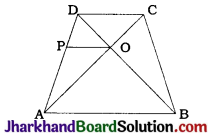Construction: Through O draw a line parallel to AB which intersects AD at P.
Proof: Here, OP || AB and AB || DC.
∴ OP || DC.
In ΔADC, OP || DC and points P and O lie on AD and AC respectively.
∴ $$\frac{OA}{OC}=\frac{PA}{PD}$$ (Theorem 6.1) …………….(1)
In ΔDAB, OP || AB and points P and O lie on AD and BD respectively.
∴ $$\frac{PA}{PD}=\frac{OB}{OD}$$ (Theorem 6.1) …………….(2)
From (1) and (2), we get
$$\frac{OA}{OC}=\frac{OB}{OD}$$
∴ $$\frac{OA}{OB}=\frac{OC}{OD}$$
∴ $$\frac{AO}{BO}=\frac{CO}{DO}$$

Question 10.
The diagonals of a quadrilateral ABCD intersect each other at the point O such that $$\frac{AO}{BO}=\frac{CO}{DO}$$. Show that ABCD is a trapezium.
Given: In quadrilateral ABCD, diagonals AC and BD intersect at O such that $$\frac{AO}{BO}=\frac{CO}{DO}$$
To prove : ABCD is a trapezium.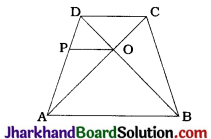Construction: Through O draw a line parallel to AB to intersect AD at P.
Proof: Here, in ΔDAB, points P and O lie on DA and DB respectively and PO || AB.
∴ $$\frac{AP}{DP}=\frac{BO}{DO}$$ (Theorem 6.1) ………….(1)
Now, $$\frac{AO}{BO}=\frac{CO}{DO}$$ (Given)
∴ $$\frac{AO}{CO}=\frac{BO}{DO}$$ ………….(2)
From (1) and (2),
$$\frac{AP}{DP}=\frac{AO}{CO}$$
Now, in ΔADC, points P and O lie on AD and AC respectively and $$\frac{AP}{DP}=\frac{AO}{CO}$$
∴ By theorem 6.2. PO || DC.
Now, PO || AB (By construction) and PO || DC (Proved).
Hence, AB || DC
Thus, in quadrilateral ABCD, AB || DC.
Hence, ABCD is a trapezium.